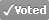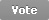1## Electromagnetism

 By: Sean Pearce (School of Physics and Astronomy) Competition Year: 2017 Votes (1) | Comments (0) < Previous     Next >
To make this research consistent with notation,
to the electric field, I will give the designation,
the force per unit charge E,
and to the magnetic field B.

From Faraday and Lenz I could see the fact
that to a closed circuit a current would act,
provided it was placed in a changing magnetic field.
An opposing current the emf would yield.

From this and with a bit of manipulation,
Stokes’ law gives us the equation
that the curl of the electric field
is equal to minus the rate of change of the magnetic field.

For the divergence of E I look to Gauss.
His law states that the charges housed
in a Gaussian surface is proportional to
the total flux of E on its edge going through.

Magnetic monopoles have not been discovered
and so the null divergence of B can be recovered
by comparing it to the electric case,
noting in a closed surface that zero total charge is encased.

Now Ampère was kind enough to start an equation
showing that the curl of B has a proportional relation
to the current density vector field.
Fortunately for me, to him the full equation was concealed.

Ampère’s law is a bit of a problem
because it implies a lack of charge conservation.
I therefore propose a simple addition:
the “displacement current”, derived from my mathematical wisdom.

An amazing feat but I wont stop there.
Hold on to your seats! I’m walking on air!
With these equations it is easy to calculate
that in a vacuum a wave can propagate!

Let there be light.
Or so God would say.

Behold I am the master of the two greatest forces!
Bow to my intellectual power in your Electromagnetic courses.
My name will be remembered from heaven to hell.
You will never forget me, James Clerk Maxwell.

Functional Error 7